# Top 165 CAT Geometry Questions With Solutions

Geometry is an important and challenging topic in the CAT exam with a lot of weightage. CAT Geometry questions are asked from topics including Triangles, Circles, Quadrilaterals, Polygons and so on. To perform Geometry, one must thoroughly understand concepts, and formulas and gain problem-solving skills. Previous years' question papers are invaluable resources for CAT preparation, especially for mastering the CAT Quantitative Aptitude section. By solving the questions from CAT's previous papers, candidates can understand the types of questions asked, the level of difficulty, and the exam pattern. Below, you can find all those Geometry questions separated year-wise, along with the video solution for each question. Also, you can download all the below questions in a PDF format consisting of video solutions for every problem explained by the CAT experts. Click the link below to download the CAT Geometry Questions with video Solutions PDF.

## CAT Geometry Topic-wise Weigthage Over Past 5 Years

 Topic 2022 2021 2020 2019 2018 Triangles 4 2 2 2 3 Circles 1 1 2 3 4 Quadrilaterals 3 5 5 0 5 Polygons 1 2 0 2 0 Mensuration 0 0 1 3 1 Coordinate Geometry 0 0 0 1 0 Total 9 10 10 11 13

## CAT Geometry Formulas PDF

CAT Geometry is one of the most important topics in the quantitative aptitude section, and it is vital to have a clear understanding of the formulas related to them. As mentioned earlier, there will be high weightage for this concept if you can compare the past few CAT question papers. To help the aspirants to ace this topic, we have made a PDF containing a comprehensive list of formulas, tips, and tricks that you can use to solve Geometry questions with ease and speed. Click on the below link to download the CAT Geometry formulas PDF.

## CAT 2022 Geometry questions

#### Question 1

In triangle ABC, altitudes AD and BE are drawn to the corresponding bases. If $$\angle BAC = 45^{\circ}$$ and $$\angle ABC=\theta\$$, then $$\frac{AD}{BE}$$ equals

#### Question 2

Suppose the medians BD and CE of a triangle ABC intersect at a point O. If area of triangle ABC is 108 sq. cm., then, the area of the triangle EOD, in sq. cm., is

#### Question 3

A trapezium $$ABCD$$ has side $$AD$$ parallel to $$BC, \angle BAD = 90^\circ, BC = 3$$ cm and $$AD= 8$$ cm. If the perimeter of this trapezium is 36 cm, then its area, in sq. cm, is

#### Question 4

Regular polygons A and B have number of sides in the ratio 1 : 2 and interior angles in the ratio 3 : 4. Then the number of sides of B equals

#### Question 5

The lengths of all four sides of a quadrilateral are integer valued. If three of its sides are of length 1 cm, 2 cm and 4 cm, then the total number of possible lengths of the fourth side is

#### Question 6

All the vertices of a rectangle lie on a circle of radius R. If the perimeter of the rectangle is P, then the area of the rectangle is

#### Question 7

In a triangle ABC, AB = AC = 8 cm. A circle drawn with BC as diameter passes through A. Another circle drawn with center at A passes through Band C. Then the area, in sq. cm, of the overlapping region between the two circles is

#### Question 8

Let ABCD be a parallelogram such that the coordinates of its three vertices A, B, C are (1, 1), (3, 4) and (−2, 8), respectively. Then, the coordinates of the vertex D are

#### Question 9

The length of each side of an equilateral triangle ABC is 3 cm. Let D be a point on BC such that the area of triangle ADC is half the area of triangle ABD. Then the length of AD, in cm, is

## CAT 2021 Geometry questions

#### Question 1

If the area of a regular hexagon is equal to the area of an equilateral triangle of side 12 cm, then the length, in cm, of each side of the hexagon is

#### Question 2

Suppose the length of each side of a regular hexagon ABCDEF is 2 cm.It T is the mid point of CD,then the length of AT, in cm, is

#### Question 3

If a rhombus has area 12 sq cm and side length 5 cm, then the length, in cm, of its longer diagonal is

#### Question 4

The cost of fencing a rectangular plot is ₹ 200 per ft along one side, and ₹ 100 per ft along the three other sides. If the area of the rectangular plot is 60000 sq. ft, then the lowest possible cost of fencing all four sides, in INR, is

#### Question 5

A park is shaped like a rhombus and has area 96 sq m. If 40 m of fencing is needed to enclose the park, the cost, in INR, of laying electric wires along its two diagonals, at the rate of ₹125 per m, is

#### Question 6

The sides AB and CD of a trapezium ABCD are parallel, with AB being the smaller side. P is the midpoint of CD and ABPD is a parallelogram. If the difference between the areas of the parallelogram ABPD and the triangle BPC is 10 sq cm, then the area, in sq cm, of the trapezium ABCD is

#### Question 7

A circle of diameter 8 inches is inscribed in a triangle ABC where $$\angle ABC = 90^\circ$$. If BC = 10 inches then the area of the triangle in square inches is

#### Question 8

If a triangle ABC, $$\angle BCA=50^{0}$$. D and E are points on $$AB$$ and $$AC$$, respectively, such that $$AD=DE$$. If F is a point on $$BC$$ such that $$BD=DF$$, then $$\angle FDE$$, in degrees, is equal to

#### Question 9

Let D and E be points on sides AB and AC, respectively, of a triangle ABC, such that AD : BD = 2 : 1 and AE : CE = 2 : 3. If the area of the triangle ADE is 8 sq cm, then the area of the triangle ABC, in sq cm, is

#### Question 10

Let ABCD be a parallelogram. The lengths of the side AD and the diogonal AC are 10cm and 20cm, respectively. If the angle $$\angle ADC$$ is equal to $$30^{0}$$ then the area of the parallelogram, in sq.cm is

## CAT 2020 Geometry questions

#### Question 1

Let C be a circle of radius 5 meters having center at O. Let PQ be a chord of C that passes through points A and B where A is located 4 meters north of O and B is located 3 meters east of O. Then, the length of PQ, in meters, is nearest to

#### Question 2

A solid right circular cone of height 27 cm is cut into two pieces along a plane parallel to its base at a height of 18 cm from the base. If the difference in volume of the two pieces is 225 cc, the volume, in cc, of the original cone is

#### Question 3

On a rectangular metal sheet of area 135 sq in, a circle is painted such that the circle touches two opposite sides. If the area of the sheet left unpainted is two-thirds of the painted area then the perimeter of the rectangle in inches is

#### Question 4

The sum of the perimeters of an equilateral triangle and a rectangle is 90cm. The area, T, of the triangle and the area, R, of the rectangle, both in sq cm, satisfying the relationship $$R=T^{2}$$. If the sides of the rectangle are in the ratio 1:3, then the length, in cm, of the longer side of the rectangle, is

#### Question 5

A circle is inscribed in a rhombus with diagonals 12 cm and 16 cm. The ratio of the area of circle to the area of rhombus is

#### Question 6

Let C1 and C2 be concentric circles such that the diameter of C1 is 2cm longer than that of C2. If a chord of C1 has length 6cm and is a tangent to C2, then the diameter, in cm, of C1 is

#### Question 7

The points (2,1) and (-3,-4) are opposite vertices of a parallelogram.If the other two vertices lie on the line $$x+9y+c=0$$, then c is

#### Question 8

From an interior point of an equilateral triangle, perpendiculars are drawn on all three sides. The sum of the lengths of the three perpendiculars is s. Then the area of the triangle is

#### Question 9

In a trapezium $$ABCD$$, $$AB$$ is parallel to $$DC$$, $$BC$$ is perpendicular to $$DC$$ and $$\angle BAD=45^{0}$$. If $$DC$$ = 5cm, $$BC$$ = 4 cm,the area of the trapezium in sq cm is

#### Question 10

The vertices of a triangle are (0,0), (4,0) and (3,9). The area of the circle passing through these three points is

## CAT 2019 Geometry questions

#### Question 1

Let T be the triangle formed by the straight line 3x + 5y - 45 = 0 and the coordinate axes. Let the circumcircle of T have radius of length L, measured in the same unit as the coordinate axes. Then, the integer closest to L is

#### Question 2

AB is a diameter of a circle of radius 5 cm. Let P and Q be two points on the circle so that the length of PB is 6 cm, and the length of AP is twice that of AQ. Then the length, in cm, of QB is nearest to

#### Question 3

Two circles, each of radius 4 cm, touch externally. Each of these two circles is touched externally by a third circle. If these three circles have a common tangent, then the radius of the third circle, in cm, is

#### Question 4

The base of a regular pyramid is a square and each of the other four sides is an equilateral triangle, length of each side being 20 cm. The vertical height of the pyramid, in cm, is

#### Question 5

Let ABC be a right-angled triangle with hypotenuse BC of length 20 cm. If AP is perpendicular on BC, then the maximum possible length of AP, in cm, is

#### Question 6

A man makes complete use of 405 cc of iron, 783 cc of aluminium, and 351 cc of copper to make a number of solid right circular cylinders of each type of metal. These cylinders have the same volume and each of these has radius 3 cm. If the total number of cylinders is to be kept at a minimum, then the total surface area of all these cylinders, in sq cm, is

#### Question 7

In a circle of radius 11 cm, CD is a diameter and AB is a chord of length 20.5 cm. If AB and CD intersect at a point E inside the circle and CE has length 7 cm, then the difference of the lengths of BE and AE, in cm, is

#### Question 8

In a triangle ABC, medians AD and BE are perpendicular to each other, and have lengths 12 cm and 9 cm, respectively. Then, the area of triangle ABC, in sq cm, is

#### Question 9

Corners are cut off from an equilateral triangle T to produce a regular hexagon H. Then, the ratio of the area of H to the area of T is

#### Question 10

Let A and B be two regular polygons having a and b sides, respectively. If b = 2a and each interior angle of B is $$\frac{3}{2}$$ times each interior angle of A, then each interior angle, in degrees, of a regular polygon with a + b sides is

#### Question 11

If the rectangular faces of a brick have their diagonals in the ratio $$3 : 2 \surd3 : \surd{15}$$, then the ratio of the length of the shortest edge of the brick to that of its longest edge is

## CAT 2018 Geometry questions

#### Question 1

In a circle, two parallel chords on the same side of a diameter have lengths 4 cm and 6 cm. If the distance between these chords is 1 cm, then the radius of the circle, in cm, is

#### Question 2

Let ABCD be a rectangle inscribed in a circle of radius 13 cm. Which one of the following pairs can represent, in cm, the possible length and breadth of ABCD?

#### Question 3

Points E, F, G, H lie on the sides AB, BC, CD, and DA, respectively, of a square ABCD. If EFGH is also a square whose area is 62.5% of that of ABCD and CG is longer than EB, then the ratio of length of EB to that of CG is

#### Question 4

A parallelogram ABCD has area 48 sqcm. If the length of CD is 8 cm and that of AD is s cm, then which one of the following is necessarily true?

#### Question 5

A chord of length 5 cm subtends an angle of 60° at the centre of a circle. The length, in cm, of a chord that subtends an angle of 120° at the centre of the same circle is

#### Question 6

Given an equilateral triangle T1 with side 24 cm, a second triangle T2 is formed by joining the midpoints of the sides of T1. Then a third triangle T3 is formed by joining the midpoints of the sides of T2. If this process of forming triangles is continued, the sum of the areas, in sq cm, of infinitely many such triangles T1, T2, T3,... will be

#### Question 7

A right circular cone, of height 12 ft, stands on its base which has diameter 8 ft. The tip of the cone is cut off with a plane which is parallel to the base and 9 ft from the base. With $$\pi$$ = 22/7, the volume, in cubic ft, of the remaining part of the cone is

#### Question 8

The area of a rectangle and the square of its perimeter are in the ratio 1 ∶ 25. Then the lengths of the shorter and longer sides of the rectangle are in the ratio

#### Question 9

On a triangle ABC, a circle with diameter BC is drawn, intersecting AB and AC at points P and Q, respectively. If the lengths of AB, AC, and CP are 30 cm, 25 cm, and 20 cm respectively, then the length of BQ, in cm, is

#### Question 10

A triangle ABC has area 32 sq units and its side BC, of length 8 units, lies on the line x = 4. Then the shortest possible distance between A and the point (0,0) is

#### Question 11

From a rectangle ABCD of area 768 sq cm, a semicircular part with diameter AB and area 72π sq cm is removed. The perimeter of the leftover portion, in cm, is

#### Question 12

In a parallelogram ABCD of area 72 sq cm, the sides CD and AD have lengths 9 cm and 16 cm, respectively. Let P be a point on CD such that AP is perpendicular to CD. Then the area, in sq cm, of triangle APD is

#### Question 13

In a circle with center O and radius 1 cm, an arc AB makes an angle 60 degrees at O. Let R be the region bounded by the radii OA, OB and the arc AB. If C and D are two points on OA and OB, respectively, such that OC = OD and the area of triangle OCD is half that of R, then the length of OC, in cm, is

## CAT 2017 Geometry questions

#### Question 1

Let ABCDEF be a regular hexagon with each side of length 1 cm. The area (in sq cm) of a square with AC as one side is

#### Question 2

The area of the closed region bounded by the equation I x I + I y I = 2 in the two-dimensional plane is

#### Question 3

From a triangle ABC with sides of lengths 40 ft, 25 ft and 35 ft, a triangular portion GBC is cut off where G is the centroid of ABC. The area, in sq ft, of the remaining portion of triangle ABC is

#### Question 4

The base of a vertical pillar with uniform cross section is a trapezium whose parallel sides are of lengths 10 cm and 20 cm while the other two sides are of equal length. The perpendicular distance between the parallel sides of the trapezium is 12 cm. If the height of the pillar is 20 cm, then the total area, in sq cm, of all six surfaces of the pillar is

#### Question 5

Let ABC be a right-angled isosceles triangle with hypotenuse BC. Let BQC be a semi-circle, away from A, with diameter BC. Let BPC be an arc of a circle centered at A and lying between BC and BQC. If AB has length 6 cm then the area, in sq cm, of the region enclosed by BPC and BQC is

#### Question 6

The points (2, 5) and (6, 3) are two end points of a diagonal of a rectangle. If the other diagonal has the equation y =3x+c,then c is

#### Question 7

A solid metallic cube is melted to form five solid cubes whose volumes are in the ratio 1 : 1 : 8 : 27 : 27. The percentage by which the sum of the surface areas of these five cubes exceeds the surface area of the original cube is nearest to

#### Question 8

ABCD is a quadrilateral inscribed in a circle with centre O such that O lies inside the quadrilateral. If $$\angle COD = 120$$ degrees and $$\angle BAC = 30$$ degrees, then the value of $$\angle BCD$$ (in degrees) is

#### Question 9

A ball of diameter 4 cm is kept on top of a hollow cylinder standing vertically. The height of the cylinder is 3 cm, while its volume is $$9\pi$$ cubic centimeters. Then the vertical distance, in cm, of the topmost point of the ball from the base of the cylinder is

#### Question 10

If three sides of a rectangular park have a total length 400 ft, then the area of the park is maximum when the length (in ft) of its longer side is

#### Question 11

Let ABC be a right-angled triangle with BC as the hypotenuse. Lengths of AB and AC are 15 km and 20 km, respectively. The minimum possible time, in minutes, required to reach the hypotenuse from A at a speed of 30 km per hour is

#### Question 12

Let P be an interior point of a right-angled isosceles triangle ABC with hypotenuse AB. If the perpendicular distance of P from each of AB,BC,and CA is $$4(\sqrt{2}-1)$$ cm,then the area, in sq cm, of the triangle ABC is

#### Question 13

The shortest distance of the point $$(\frac{1}{2},1)$$ from the curve y = I x -1I + I x + 1I is

## CAT 2008 Geometry questions

#### Question 1

In a triangle ABC, the lengths of the sides AB and AC equal 17.5 cm and 9 cm respectively. Let D be a point on the line segment BC such that AD is perpendicular to BC. If AD = 3 cm, then what is the radius (in cm) of the circle circumscribing the triangle ABC?

#### Question 2

Consider obtuse-angled triangles with sides 8 cm, 15 cm and x cm. If x is an integer then how many such triangles exist?

#### Question 3

Consider a square ABCD with midpoints E, F, G, H of AB, BC, CD and DA respectively. Let L denote the line passing through F and H. Consider points P and Q, on L and inside ABCD such that the angles APD and BQC both equal 120°. What is the ratio of the area of ABQCDP to the remaining area inside ABCD?

#### Question 4

Two circles, both of radii 1 cm, intersect such that the circumference of each one passes through the centre of the other. What is the area (in sq. cm.) of the intersecting region?

#### Question 5

Consider a right circular cone of base radius 4 cm and height 10 cm. A cylinder is to be placed inside the cone with one of the flat surfaces resting on the base of the cone. Find the largest possible total surface area (in sq. cm) of the cylinder.

## CAT 2007 Geometry questions

#### Question 1

Two circles with centres P and Q cut each other at two distinct points A and B. The circles have the same radii and neither P nor Q falls within the intersection of the circles. What is the smallest range that includes all possible values of the angle AQP in degrees?

## CAT 2006 Geometry questions

#### Question 1

A semi-circle is drawn with AB as its diameter. From C, a point on AB, a line perpendicular to AB is drawn meeting the circumference of the semi-circle at D. Given that AC = 2 cm and CD = 6 cm, the area of the semi-circle (in sq. cm) will be:

#### Question 2

An equilateral triangle DOC is drawn inside a square ABCD. What is the value of the angle AOB in degrees?

#### Question 3

The length, breadth and height of a room are in the ratio 3:2:1. If the breadth and height are halved while the length is doubled, then the total area of the four walls of the room will

Instruction for set 1:

A punching machine is used to punch a circular hole of diameter two Units from a square sheet of aluminium of width 2 units, as shown below. The hole is punched such that the circular hole touches one corner P of the square sheet and the diameter of the hole originating at P is in line with a diagonal of the square: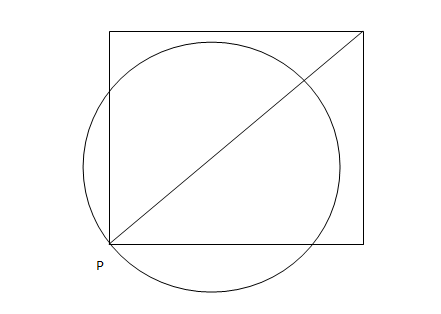#### Question 4

The proportion of the sheet area that remains after punching is:

Instruction for set 1:

A punching machine is used to punch a circular hole of diameter two Units from a square sheet of aluminium of width 2 units, as shown below. The hole is punched such that the circular hole touches one corner P of the square sheet and the diameter of the hole originating at P is in line with a diagonal of the square:#### Question 5

Find the area of the part of the circle (round punch) falling outside the square sheet.

## CAT 2005 Geometry questions

#### Question 1

Consider a triangle drawn on the X-Y plane with its three vertices at (41, 0), (0, 41) and (0, 0), each vertex being represented by its (X,Y) coordinates. The number of points with integer coordinates inside the triangle (excluding all the points on the boundary) is

#### Question 2

Four points A, B, C, and D lie on a straight line in the X-Y plane, such that AB = BC = CD, and the length of AB is 1 metre. An ant at A wants to reach a sugar particle at D. But there are insect repellents kept at points B and C. The ant would not go within one metre of any insect repellent. The minimum distance in metres the ant must traverse to reach the sugar particle is

#### Question 3

Rectangular tiles each of size 70 cm by 30 cm must be laid horizontally on a rectangular floor of size 110 cm by 130 cm, such that the tiles do not overlap. A tile can be placed in any orientation so long as its edges are parallel to the edges of the floor. No tile should overshoot any edge of the floor. The maximum number of tiles that can be accommodated on the floor is

#### Question 4

In the following figure, the diameter of the circle is 3 cm. AB and MN are two diameters such that MN is perpendicular to AB. In addition, CG is perpendicular to AB such that AE:EB = 1:2, and DF is perpendicular to MN such that NL:LM = 1:2. The length of DH in cm is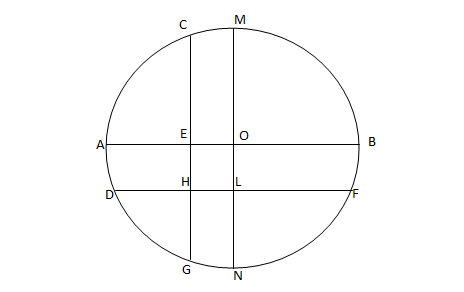#### Question 5

Consider the triangle ABC shown in the following figure where BC = 12 cm, DB = 9 cm, CD = 6 and $$\angle{BCD} = \angle{BAC}$$

What is the ratio of the perimeter of the triangle ADC to that of the triangle BDC?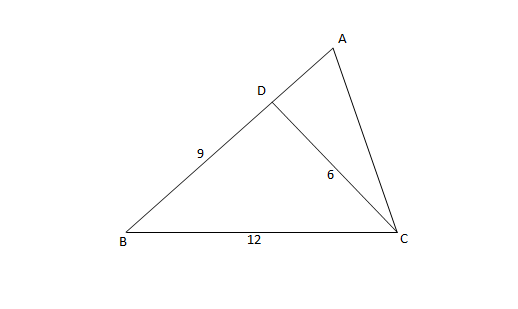#### Question 6

P, Q, S, and R are points on the circumference of a circle of radius r, such that PQR is an equilateral triangle and PS is a diameter of the circle. What is the perimeter of the quadrilateral PQSR?

#### Question 7

What is the distance in cm between two parallel chords of lengths 32 cm and 24 cm in a circle of radius 20 cm?

#### Question 8

Two identical circles intersect so that their centres, and the points at which they intersect, form a square of side 1 cm. The area in sq. cm of the portion that is common to the two circles is

#### Question 9

A jogging park has two identical circular tracks touching each other, and a rectangular track enclosing the two circles. The edges of the rectangles are tangential to the circles. Two friends, A and B, start jogging simultaneously from the point where one of the circular tracks touches the smaller side of the rectangular track. A jogs along the rectangular track, while B jogs along the two circular tracks in a figure of eight. Approximately, how much faster than A does B have to run, so that they take the same time to return to their starting point?

## CAT 2004 Geometry questions

#### Question 1

A father and his son are waiting at a bus stop in the evening. There is a lamp post behind them. The lamp post, the father and his son stand on the same straight line. The father observes that the shadows of his head and his son's head are incident at the same point on the ground. If the heights of the lamp post, the father and his son are 6 metres, 1.8 metres and 0.9 metres respectively, and the father is standing 2.1 metres away from the post then how far (in metres) is son standing form his father?

#### Question 2

Let $$C$$ be a circle with centre $$P_0$$ and $$AB$$ be a diameter of $$C$$. Suppose $$P_1$$ is the mid point of the line segment $$P_0B$$,$$P_2$$ is the mid point of the line segment $$P_1B$$ and so on. Let $$C_1,C_2,C_3,...$$ be circles with diameters $$P_0P_1, P_1P_2, P_2P_3...$$ respectively. Suppose the circles $$C_1, C_2, C_3,...$$ are all shaded. The ratio of the area of the unshaded portion of $$C$$ to that of the original circle is

#### Question 3

If the lengths of diagonals DF, AG and CE of the cube shown in the adjoining figure are equal to the three sides of a triangle, then the radius of the circle circumscribing that triangle will be?#### Question 4

On a semicircle with diameter AD, chord BC is parallel to the diameter. Further; AD and BC are separated by 2cm, while AD has length 8. What is the length of BC?

#### Question 5

A circle with radius 2 is placed against a right angle. Another smaller circle is also placed as shown in the adjoining figure. What is the radius of the smaller circle?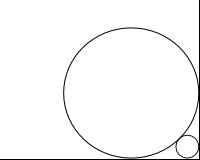#### Question 6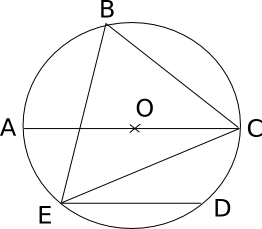In the adjoining figure, chord ED is parallel to the diameter AC of the circle. If angle CBE = 65°, then what is the value of angle DEC?

#### Question 7

A rectangular sheet of paper, when halved by folding it at the mid point of its longer side, results in a rectangle, whose longer and shorter sides are in the same proportion as the longer and shorter sides of the original rectangle. If the shorter side of the original rectangle is 2, what is the area of the smaller rectangle?

[CAT 2004]

Instruction for set 1:

Directions for the following three questions: Answer the questions on the basis of the information given below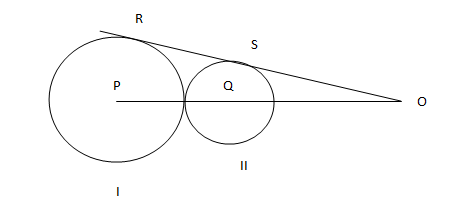In the adjoining figure I and II are circles with centres P and Q respectively, The two circles touch each other and have common tangent that touches them at points R and S respectively. This common tangent meets the line joining P and Q at O. The diameters of I and II are in the ratio 4: 3. It is also known that the length of PO is 28 cm.

#### Question 8

What is the ratio of the length of PQ to that of QO?

[CAT 2004]

Instruction for set 1:

Directions for the following three questions: Answer the questions on the basis of the information given belowIn the adjoining figure I and II are circles with centres P and Q respectively, The two circles touch each other and have common tangent that touches them at points R and S respectively. This common tangent meets the line joining P and Q at O. The diameters of I and II are in the ratio 4: 3. It is also known that the length of PO is 28 cm.

#### Question 9

What is the radius of the circle II?

[CAT 2004]

Instruction for set 1:

Directions for the following three questions: Answer the questions on the basis of the information given belowIn the adjoining figure I and II are circles with centres P and Q respectively, The two circles touch each other and have common tangent that touches them at points R and S respectively. This common tangent meets the line joining P and Q at O. The diameters of I and II are in the ratio 4: 3. It is also known that the length of PO is 28 cm.

#### Question 10

The length of SO is

[CAT 2004]

## CAT 2003 Geometry questions

#### Question 1

Each side of a given polygon is parallel to either the X or the Y axis. A corner of such a polygon is said to be convex if the internal angle is 90° or concave if the internal angle is 270°. If the number of convex corners in such a polygon is 25, the number of concave corners must be

#### Question 2

There are two concentric circles such that the area of the outer circle is four times the area of the inner circle. Let A, B and C be three distinct points on the perimeter of the outer circle such that AB and AC are tangents to the inner circle. If the area of the outer circle is 12 square centimeters then the area (in square centimeters) of the triangle ABC would be

#### Question 3

Three horses are grazing within a semi-circular field. In the diagram given below, AB is the diameter of the semi-circular field with center at O. Horses are tied up at P, R and S such that PO and RO are the radii of semi-circles with centers at P and R respectively, and S is the center of the circle touching the two semi-circles with diameters AO and OB. The horses tied at P and R can graze within the respective semi-circles and the horse tied at S can graze within the circle centred at S. The percentage of the area of the semi-circle with diameter AB that cannot be grazed by the horses is nearest to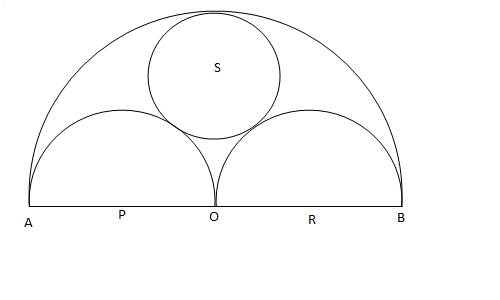#### Question 4

In the figure below, ABCDEF is a regular hexagon and $$\angle{AOF}$$ = 90° . FO is parallel to ED. What is the ratio of the area of the triangle AOF to that of the hexagon ABCDEF?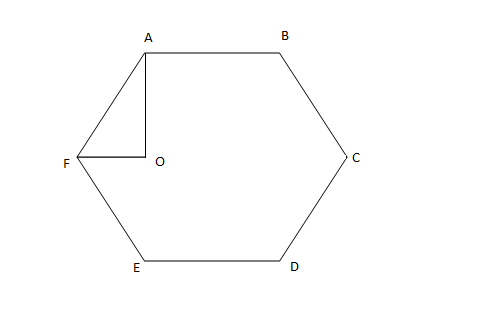#### Question 5

A vertical tower OP stands at the center O of a square ABCD. Let h and b denote the length OP and AB respectively. Suppose $$\angle{APB}$$ = 60° then the relationship between h and b can be expressed as

#### Question 6

In the triangle ABC, AB = 6, BC = 8 and AC = 10. A perpendicular dropped from B, meets the side AC at D. A circle of radius BD (with center B) is drawn. If the circle cuts AB and BC at P and Q respectively, the AP:QC is equal to

#### Question 7

In the diagram given below, $$\angle{ABD}$$ = $$\angle{CDB}$$ = $$\angle{PQD}$$ = 90° . If AB:CD = 3:1, the ratio of CD: PQ is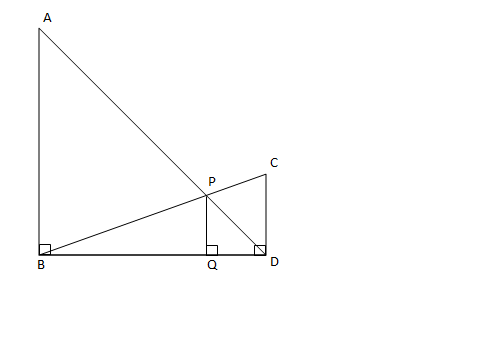#### Question 8

In the figure below, AB is the chord of a circle with center O. AB is extended to C such that BC = OB. The straight line CO is produced to meet the circle at D. If $$\angle{ACD}$$ = y degrees and $$\angle{AOD}$$ = x degrees such that x = ky, then the value of k is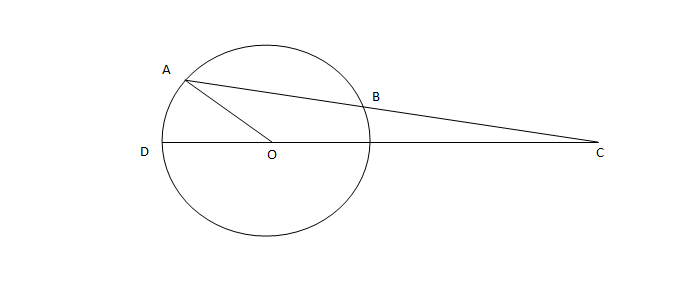#### Question 9

In the figure below, the rectangle at the corner measures 10 cm × 20 cm. The corner A of the rectangle is also a point on the circumference of the circle. What is the radius of the circle in cm?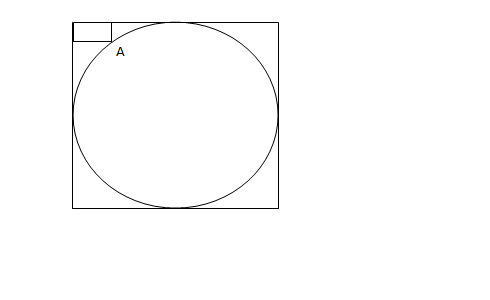## CAT 2002 Geometry questions

#### Question 1

The length of the common chord of two circles of radii 15 cm and 20 cm, whose centres are 25 cm apart, is

#### Question 2

Four horses are tethered at four corners of a square plot of side 14 m so that the adjacent horses can just reach one another. There is a small circular pond of area 20 $$m^2$$ at the centre. Find the ungrazed area.

#### Question 3

On a straight road XY, 100 m long, five heavy stones are placed 2 m apart beginning at the end X. A worker, starting at X, has to transport all the stones to Y, by carrying only one stone at a time. The minimum distance he has to travel is

#### Question 4

In the figure given below, ABCD is a rectangle. The area of the isosceles right triangle ABE = 7 $$cm^2$$ ; EC = 3(BE). The area of ABCD (in $$cm^2$$) is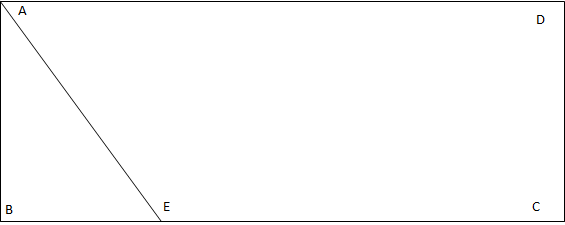#### Question 5

The area of the triangle whose vertices are (a,a), (a + 1, a + 1) and (a + 2, a) is

[CAT 2002]

#### Question 6

Instead of walking along two adjacent sides of a rectangular field, a boy took a short cut along the diagonal and saved a distance equal to half the longer side. Then the ratio of the shorter side to the longer side is

#### Question 7

Neeraj has agreed to mow a lawn, which is a 20 m × 40 m rectangle. He mows it with 1 m wide strip. If Neeraj starts at one corner and mows around the lawn toward the centre, about how many times would he go round before he has mowed half the lawn? (Round off the answer to two decimal digits)

#### Question 8

In the following figure, ACB is a right-angled triangle. AD is the altitude. Circles are inscribed within the triangle ACD and triangle BCD. P and Q are the centers of the circles. The distance PQ is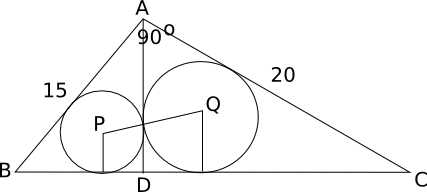The length of AB is 15 m and AC is 20 m

#### Question 9

In triangle ABC, the internal bisector of $$\angle{A}$$ meets BC at D. If AB = 4, AC = 3 and $$\angle{A}$$ = 60° , then the length of AD is

Instruction for set 1:

Directions for the next two questions: Answer the questions based on the following diagram

In the following diagram, $$\angle{ABC}$$ = 90° = $$\angle{DCH}$$ = $$\angle{DOE}$$ = $$\angle{EHK}$$ = $$\angle{FKL}$$ = $$\angle{GLM}$$ = $$\angle{LMN}$$

AB = BC = 2CH = 2CD = EH = FK = 2HK = 4KL = 2LM = MN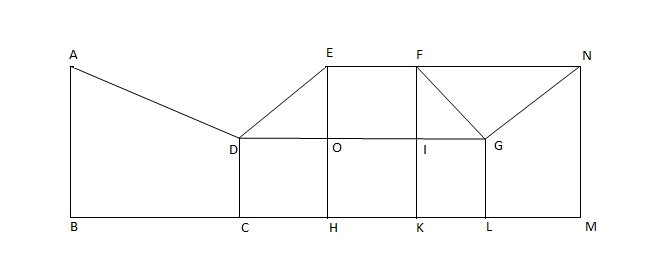#### Question 10

The magnitude of $$\angle{FGO}$$ =

Instruction for set 1:

Directions for the next two questions: Answer the questions based on the following diagram

In the following diagram, $$\angle{ABC}$$ = 90° = $$\angle{DCH}$$ = $$\angle{DOE}$$ = $$\angle{EHK}$$ = $$\angle{FKL}$$ = $$\angle{GLM}$$ = $$\angle{LMN}$$

AB = BC = 2CH = 2CD = EH = FK = 2HK = 4KL = 2LM = MN#### Question 11

What is the ratio of the areas of the two quadrilaterals ABCD to DEFG?

## CAT 2001 Geometry questions

#### Question 1

A certain city has a circular wall around it, and this wall has four gates pointing north, south, east and west. A house stands outside the city, 3 km north of the north gate, and it can just be seen from a point 9 km east of the south gate. What is the diameter of the wall that surrounds the city?

#### Question 2

In the given diagram, ABCD is a rectangle with AE = EF = FB. What is the ratio of the areas of CEF and that of the rectangle?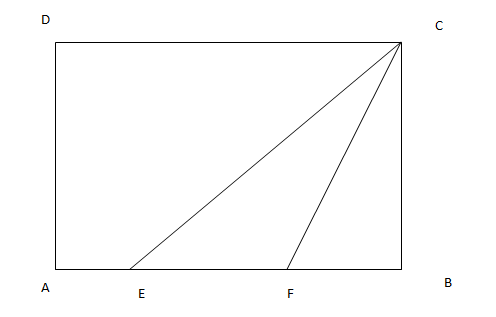#### Question 3

A ladder leans against a vertical wall. The top of the ladder is 8 m above the ground. When the bottom of the ladder is moved 2 m farther away from the wall, the top of the ladder rests against the foot of the wall. What is the length of the ladder?

#### Question 4

Two sides of a plot measure 32 m and 24 m and the angle between them is a perfect right angle. The other two sides measure 25 m each and the other three angles are not right angles. What is the area of the plot?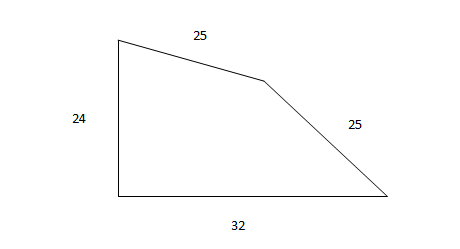#### Question 5

Euclid has a triangle in mind. Its longest side has length 20 and another of its sides has length 10. Its area is 80. What is the exact length of its third side?

#### Question 6

In triangle DEF shown below, points A, B and C are taken on DE, DF and EF respectively such that EC = AC and CF = BC. If angle D equals 40 degress , then angle ACB is ?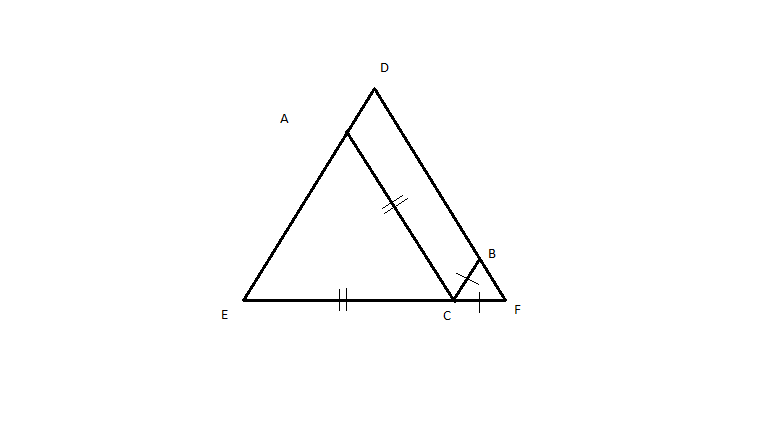#### Question 7

Based on the figure below, what is the value of x, if y = 10?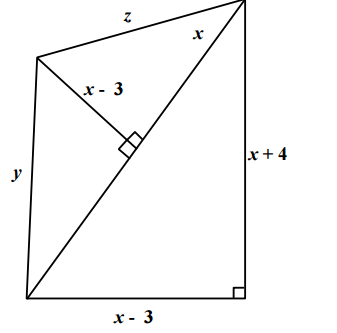#### Question 8

A rectangular pool of 20 m wide and 60 m long is surrounded by a walkway of uniform width. If the total area of the walkway is 516 $$m^2$$ , how wide, in metres, is the walkway?

#### Question 9

A square, whose side is 2 m, has its corners cut away so as to form an octagon with all sides equal. Then the length of each side of the octagon, in metres, is

## CAT 2000 Geometry questions

#### Question 1

What is the number of distinct triangles with integral valued sides and perimeter 14?

#### Question 2

ABCD is a rhombus with the diagonals AC and BD intersection at the origin on the x-y plane. The equation of the straight line AD is x + y = 1. What is the equation of BC?

#### Question 3

Consider a circle with unit radius. There are 7 adjacent sectors, S1, S2, S3,....., S7 in the circle such that their total area is (1/8)th of the area of the circle. Further, the area of the $$j^{th}$$ sector is twice that of the $$(j-1)^{th}$$ sector, for j=2, ...... 7. What is the angle, in radians, subtended by the arc of S1 at the centre of the circle?

#### Question 4

If a,b,c are the sides of a triangle, and $$a^2 + b^2 +c^2 = bc + ca + ab$$, then the triangle is:

#### Question 5

In the figure AB=BC=CD=DE=EF=FG=GA, then $$\angle{DAE}$$ is approximately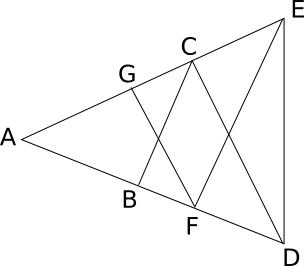#### Question 6

ABCDEFGH is a regular octagon. A and E are opposite vertices of the octagon. A frog starts jumping from vertex to vertex, beginning from A. From any vertex of the octagon except E, it may jump to either of the two adjacent vertices. When it reaches E, the frog stops and says there. Let $$a_n$$ be the number of distinct paths of exactly n jumps ending in E. Then, what is the value of $$a_{2n-1}$$?

#### Question 7

A farmer has decided to build a wire fence along one straight side of this property. For this, he planned to place several fence-posts at six metre intervals, with posts fixed at both ends of the side. After he bought the posts and wire, he found that the number of posts he had bought was five less than required. However, he discovered that the number of posts he had bought would be just sufficient if he spaced them eight metres apart. What is the length of the side of his property and how many posts did he buy?

## CAT 1999 Geometry questions

#### Question 1

The figure below shows two concentric circles with centre 0. PQRS is a square, inscribed in the outer circle. It also circumscribes the inner circle, touching it at points B, C, D and A. What is the ratio of the perimeter of the outer circle to that of polygon ABCD?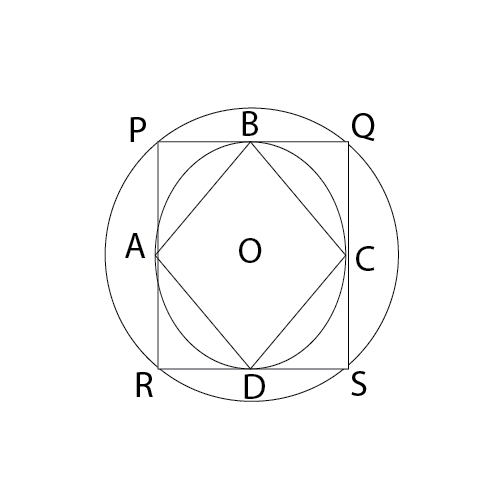#### Question 2

There is a circle of radius 1 cm. Each member of a sequence of regular polygons S1(n), n = 4,5,6,... , where n is the number of sides of the polygon, is circumscribing the circle; and each member of the sequence of regular polygons S2(n), n = 4,5,6.... where n is the number of sides of the polygon, is inscribed in the circle. Let L1(n) and L2(n) denote the perimeters of the corresponding polygons of S1(n) and S2(n). Then $$\frac{L1(13)+2\pi }{L2(17)}$$ is

#### Question 3

There is a square field with each side 500 metres long. It has a compound wall along its perimeter. At one of its comers, a triangular area of the field is to be cordoned off by erecting a straight line fence. The compound wall and the fence will form its borders. If the length of the fence is 100 metres, what is the maximum area in square metres that can be cordoned off?

#### Question 4

Ten points are marked on a straight line and eleven points are marked on another straight line. How many triangles can be constructed with vertices from among the above points?

Instruction for set 1:

DIRECTIONS for the following two questions: These questions are based on the situation given below:

A rectangle PRSU, is divided into two smaller rectangles PQTU, and QRST by the line TQ. PQ=10cm, QR = 5 cm and RS = 10 cm. Points A, B, F are within rectangle PQTU, and points C, D, E are within the rectangle QRST. The closest pair of points among the pairs (A, C), (A, D), (A, E), (F, C), (F, D), (F, E), (B, C), (B, D), (B, E) are $$10 \sqrt{3}$$ cm apart.

#### Question 5

Which of the following statements is necessarily true?

Instruction for set 1:

DIRECTIONS for the following two questions: These questions are based on the situation given below:

A rectangle PRSU, is divided into two smaller rectangles PQTU, and QRST by the line TQ. PQ=10cm, QR = 5 cm and RS = 10 cm. Points A, B, F are within rectangle PQTU, and points C, D, E are within the rectangle QRST. The closest pair of points among the pairs (A, C), (A, D), (A, E), (F, C), (F, D), (F, E), (B, C), (B, D), (B, E) are $$10 \sqrt{3}$$ cm apart.

#### Question 6

AB > AF > BF; CD > DE > CE; and BF = $$6\sqrt{5}$$ cm. Which is the closest pair of points among all the six given points?

## CAT 1998 Geometry questions

#### Question 1

A circle circumscribes a square. What is the area of the square?

I. Radius of the circle is given.

II. Length of the tangent from a point 5 cm away from the centre of the circle is given.

#### Question 2

Three circles, each of radius 20, have centres at P, Q and R. Further, AB = 5, CD = 10 and EF = 12. What is the perimeter of ΔPQR ?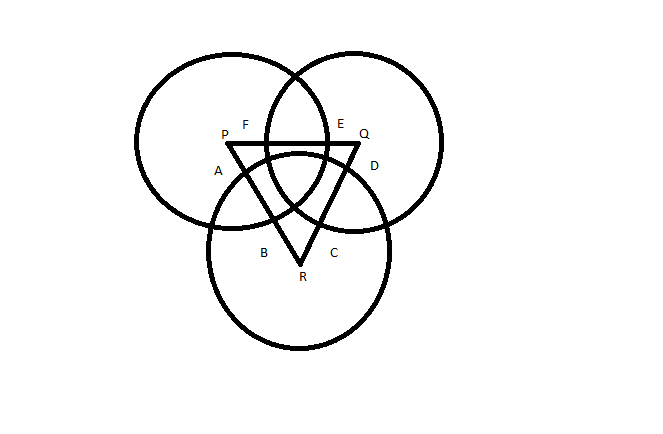#### Question 3

Four identical coins are placed in a square. For each coin the ratio of area to circumference is same as the ratio of circumference to area. Then find the area of the square that is not covered by the coins.

Instruction for set 1:

Answer the questions based on the following information. A cow is tethered at point A by a rope. Neither the rope nor the cow is allowed to enter ΔABC.
∠BAC = 30°
I(AB) = I(AC) = 10 m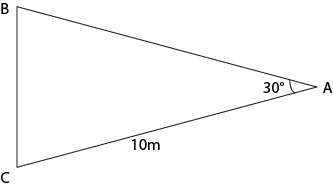#### Question 4

What is the area that can be grazed by the cow if the length of the rope is 8 m?

Instruction for set 1:

Answer the questions based on the following information. A cow is tethered at point A by a rope. Neither the rope nor the cow is allowed to enter ΔABC.
∠BAC = 30°
I(AB) = I(AC) = 10 m#### Question 5

What is the area that can be grazed by the cow if the length of the rope is 12 m?

## CAT 1997 Geometry questions

#### Question 1

The sum of the areas of two circles, which touch each other externally, is $$153\pi$$. If the sum of their radii is 15, find the ratio of the larger to the smaller radius.

#### Question 2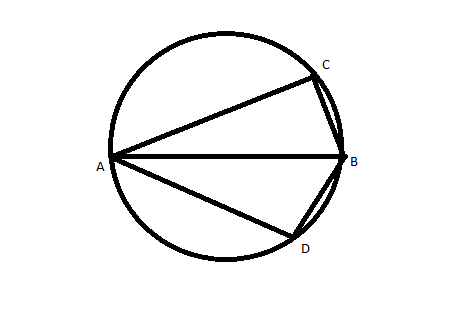AB is the diameter of the given circle, while points C and D lie on the circumference as shown. If AB is 15 cm, AC is 12 cm and BD is 9 cm, find the area of the quadrilateral ACBD.

#### Question 3

The adjoining figure shows a set of concentric squares. If the diagonal of the innermost square is 2 units, and if the distance between the corresponding corners of any two successive squares is 1 unit, find the difference between the areas of the eighth and the seventh squares, counting from the innermost square.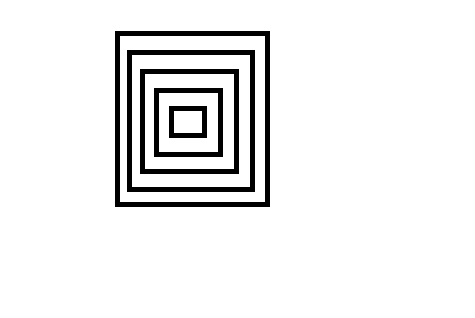#### Question 4

In ABC, points P, Q and R are the mid-points of sides AB, BC and CA respectively. If area of ABC is 20 sq. units, find the area of PQR.

#### Question 5

In a rectangle, the difference between the sum of the adjacent sides and the diagonal is half the length of the longer side. What is the ratio of the shorter to the longer side?

#### Question 6

In the adjoining figure, points A, B, C and D lie on the circle. AD = 24 and BC = 12. What is the ratio of the area of CBE to that of ADE?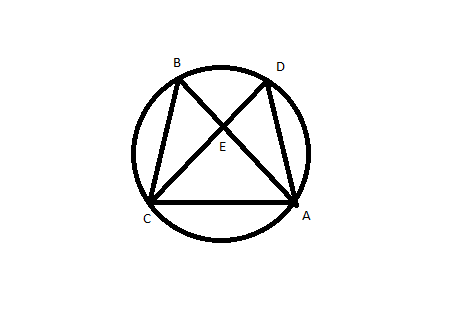#### Question 7

In the given figure, EADF is a rectangle and ABC is a triangle whose vertices lie on the sides of EADF and AE = 22, BE = 6, CF = 16 and BF = 2. Find the length of the line joining the mid-points of the sides AB and BC.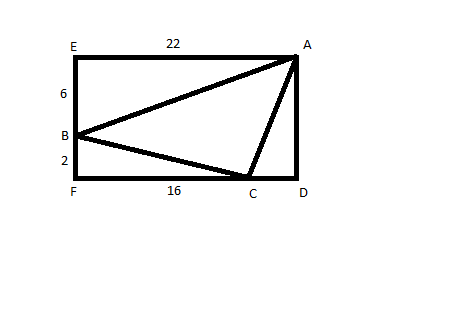## CAT 1996 Geometry questions

#### Question 1

A cube of side 12 cm is painted red on all the faces and then cut into smaller cubes, each of side 3 cm. What is the total number of smaller cubes having none of their faces painted?

#### Question 2

If ABCD is a square and BCE is an equilateral triangle, what is the measure of ∠DEC?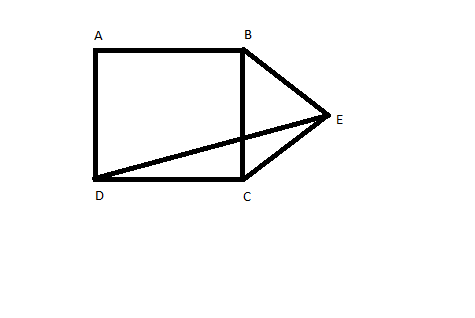#### Question 3

From a circular sheet of paper with a radius 20 cm, four circles of radius 5 cm each are cut out. What is the ratio of the uncut to the cut portion?

#### Question 4

A wooden box  of thickness 0.5 cm, length 21 cm, width 11 cm and height 6 cm is painted on the inside. The expenses of painting are Rs. 70. What is the rate of painting per square centimetres?

#### Question 5

The figure shows a circle of diameter AB and radius 6.5 cm. If chord CA is 5 cm long, find the area of triangle ABC.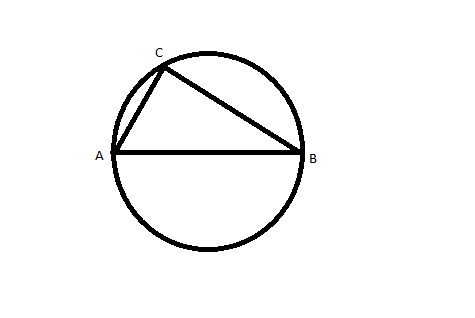#### Question 6

In triangleABC, ∠B is a right angle, AC = 6 cm, and D is the mid-point of AC. The length of BD is

#### Question 7

The figure shows the rectangle ABCD with a semicircle and a circle inscribed inside in it as shown. What is the ratio of the area of the circle to that of the semicircle?

#### Question 8

The points of intersection of three lines $$2x+3y-5=0, 5x-7y+2=0$$ and $$9x-5y-4=0$$

## CAT 1991 Geometry questions

#### Question 1

Let the consecutive vertices of a square S be A,B,C &D. Let E,F & G be the mid-points of the sides AB, BC & AD respectively of the square. Then the ratio of the area of the quadrilateral EFDG to that of the square S is nearest to

#### Question 2

A circle is inscribed in a given square and another circle is circumscribed about the square. What is the ratio of the area of the inscribed circle to that of the circumscribed circle?

#### Question 3

A square piece of cardboard of sides ten inches is taken and four equal squares pieces are removed at the corners, such that the side of this square piece is also an integer value. The sides are then turned up to form an open box. Then the maximum volume such a box can have is

#### Question 4

A one rupee coin is placed on a table. The maximum number of similar one rupee coins which can be placed on the table, around it, with each one of them touching it and only two others is

#### Question 5

A man starting at a point walks one km east, then two km north, then one km east, then one km north, then one km east and then one km north to arrive at the destination. What is the shortest distance from the starting point to the destination?

#### Question 6

What is the distance between the points A(3, 8) and B(-2,-7)?

#### Question 7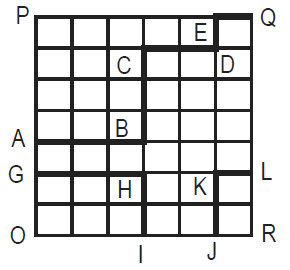What is the area under the line GHI-JKL in the given quadrilateral OPQR, knowing that all the small spaces are squares of the same area?
I. Length ABCDEQ is greater than or equal to 60.
II. Area OPQR is less than or equal to 1512.